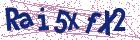# Effective numerical methods for nonlinear singular two-point boundary value Fredholm integro-differential equations

Document Type : Research Article

Author

Department of Basic Sciences, Shahid Sattari Aeronautical University of Science and Technology, P.O. Box: 13846-63113, Tehran, Iran.

Abstract

We deal with some effective numerical methods for solving a class of nonlinear singular two-point boundary value Fredholm integro-differential equations. Using an appropriate interpolation and a q-order quadrature rule of integration, the original problem will be approximated by the non-linear finite difference equations and so reduced to a nonlinear algebraic system that can be simply implemented. The convergence properties of the proposed method are discussed, and it is proved that its convergence order will be of O(hmin{ 72 ,q− 12 }). Ample numerical results are addressed to con-
firm the expected convergence order as well as the accuracy and efficiency of the proposed method.

Keywords20.1001.1.24236977.2023.13.3.5.0

Main Subjects

#### References

 Amiraliyev, G.M., Durmaz, M.E. and Kudu, M. A numerical method for a second order singularly perturbed Fredholm integro-differential equa-tion, Miskolc Math. Notes 22(1) (2021) 37–48.
 Chambre, P.L. On the solution of the Poisson-Boltzmann equation with application to the theory of thermal explosions, J. Chem. Phys. 20 (1952) 1795–1797.
 Chandrasekhar, S. Introduction to the Study of Stellar Structure, Dover, New York, 1967.
 Chen, J., He, M.F. and Huang, Y. A fast multiscale Galerkin method for solving second order linear fredholm integro-differential equation with Dirichlet boundary conditions, J. Comput. Appl. Math. 364 (2020) 112352.
 Duggan, R.C. and Goodman, A.M. Pointwise bounds for a nonlinear heat conduction model of the human head, Bull. Math. Biol. 48 (2) (1986) 229–236.
 Durmaz, M.E. and Amiraliyev, G.M. A robust numerical method for a singularly perturbed Fredholm integro-differential equation, Mediterr. J. Math. 18(1) (2021), Paper No. 24, 17 pp.
 Durmaz, M.E., Amiraliyev, G.M. and Kudu, M. Numerical solution of a singularly perturbed Fredholm integro differential equation with Robin boundary condition, Turk. J. Math. 46 (1) (2022) 207–224.
 Durmaz, M.E., Cakır, M. and Amirali, G. Parameter uniform second-order numerical approximation for the integro-differential equations in-volving boundary layers, Commun. Fac. Sci. Univ. Ank. Ser. A1 Math. Stat. 71(4) (2022) 954–967.
 Flagg, R.C., Luning, C.D. and Perry, W.L. Implementation of new it-erative techniques for solutions of Thomas-Fermi and Emden-Fowler equations, J. Comput. Phys. 38 (3) (1980) 396–405.
 Gebeyehu, M., Garoma, H. and Deressa, A. Fitted numerical method for singularly perturbed semilinear three-point boundary value problem, Iranian Journal of Numerical Analysis and Optimization, 12(1) (2022)145–162.
 Gümgüm, S. Taylor wavelet solution of linear and nonlinear Lane-Emden equations, Appl. Numer. Math. 158 (2020) 44–53.
 Khan, H. and Xu, H. Series solution to the Thomas-Fermi equation, Phys. Lett. A 365 (2007) 111–115.
 Khan, S. and Khan, A. A fourth-order method for solving singularly perturbed boundary value problems using nonpolynomial splines, Iranian Journal of Numerical Analysis and Optimization, 12 (2) (2022) 483–497.
 Khuri, S.A. and Sayfy, A. A novel approach for the solution of a class of singular boundary value problems arising in physiology, Math. Comput. Model 52 (3) (2010) 626–636.
 Kilicman, A., Hashim, I., Tavassoli Kajani, M. and Maleki, M. On the rational second kind Chebyshev pseudospectral method for the solution of the Thomas-Fermi equation over an infinite interval, J. Comput. Appl. Math. 257 (7) (2014) 79–85.
 Kumar, M., Mishra, H.K. and Singh, P. A boundary value approach for a class of linear singularly perturbed boundary value problems, Adv. Eng. Softw. 10 (2009) 298–304.
 Lima, P.M. and Morgado, L. Numerical modeling of oxygen diffusion in cells with Michaelis-Menten uptake kinetics, J. Math. Chem. 48 (2010) 145–158.
 Lin, S.H. Oxygen diffusion in a spherical cell with nonlinear oxygen uptake kinetics, J. Theor. Biol. 60 (2) (1976) 449–457.
 Mohapatra, J. and Govindarao, L. A fourth-order optimal numerical approximation and its convergence for singularly perturbed time delayed parabolic problems, Iranian Journal of Numerical Analysis and Optimiza-tion, 12 (2) (2022) 250–276.
 Priyadarshana, S., Sahu, S.R. and Mohapatra, J. Asymptotic and nu-merical methods for solving singularly perturbed differential difference equations with mixed shifts, Iranian Journal of Numerical Analysis and Optimization, 12 (1) (2022) 55–72.
 Reddy, Y.N. and Chakravarthy, P.P. An initial-value approach for solving singularly perturbed two-point boundary value problems, Appl. Math. Comput. 155 (2004) 95–110.
 Singh, O.P., Pandey, R.K. and Singh, V.K. An analytic algorithm of Lane-Emden type equations arising in astrophysics using modified Homo-topy analysis method, Comput. Phys. Commun. 180 (2009) 1116–1124.
 Woldaregay, M.M. and Duressa, G.F. Exponentially fitted tension spline method for singularly perturbed differential difference equations, Iranian Journal of Numerical Analysis and Optimization, 11 (2) (2021) 261–282.
 Zhang, F. Matrix theory, Springer, New York, 2011.